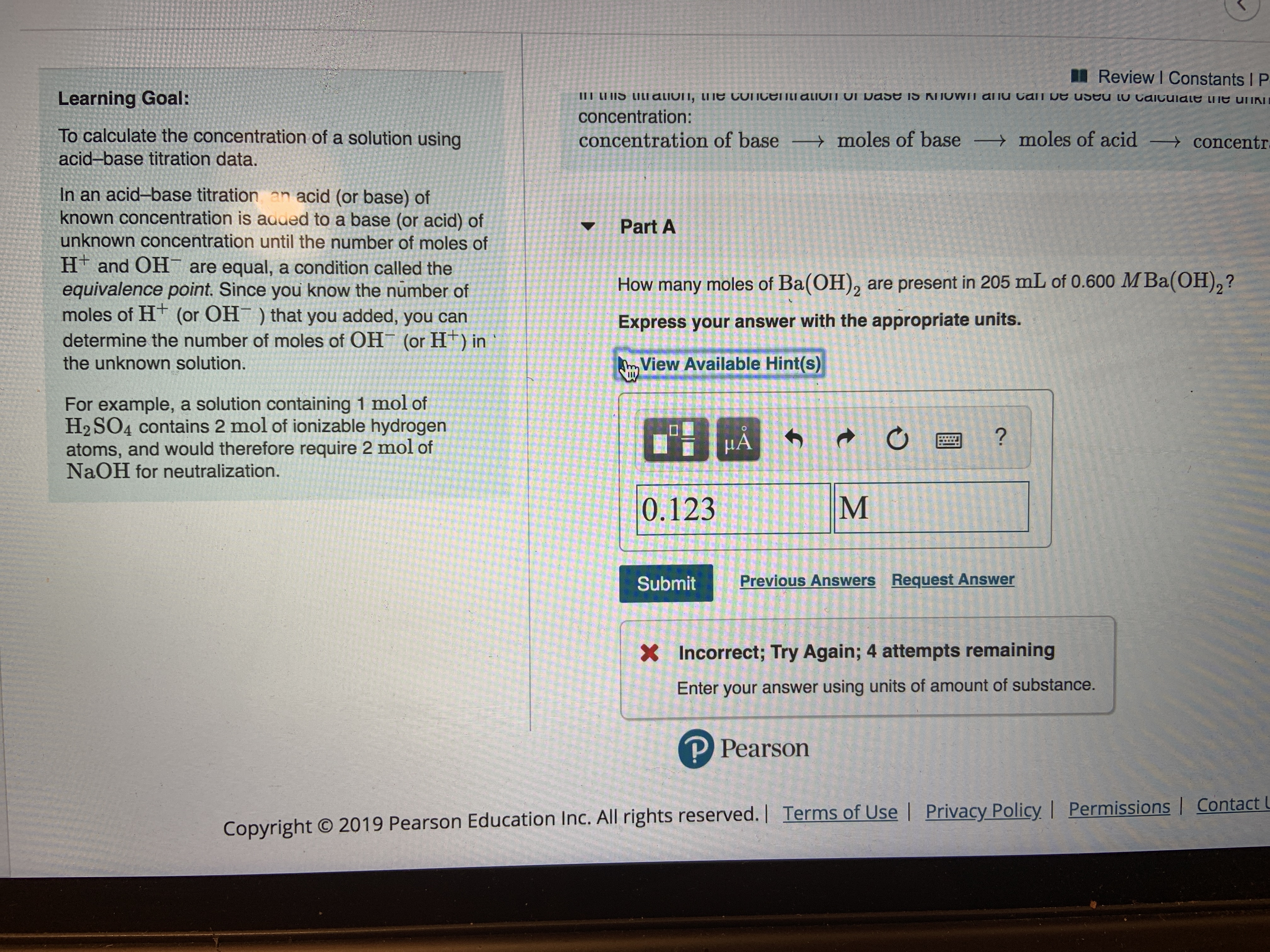# I Review I Constants I P III LI IS LILI alivin, ti e connceIILI aliun UI Dase iS KNOWN aliu Can Ue useu LU Caiculale e uiKN Learning Goal: concentration: To calculate the concentration of a solution using moles of base moles of acid concentration of base concentr acid-base titration data. In an acid-base titration an acid (or base) of known concentration is aaded to a base (or acid) of unknown concentration until the number of moles of H and OH are equal, a condition called the equivalence point. Since you know the number of moles of HT (or OH) that you added, you can Part A How many moles of Ba(OH)2 are present in 205 mL of 0.600 M Ba(OH),? Express your answer with the appropriate units. determine the number of moles of OH (or H) in View Available Hint(s) the unknown solution. For example, a solution containing 1 mol of H2 SO4 contains 2 mol of ionizable hydrogen atoms, and would therefore require 2 mol of NaOH for neutralization. A ? M 0.123 Previous Answers Request Answer Submit Incorrect; Try Again; 4 attempts remaining X Enter your answer using units of amount of substance. P Pearson Copyright O 2019 Pearson Education Inc. All rights reserved.| Terms of Use | Privacy Policy, I Permissions | Contact

Questionhelp_outlineImage TranscriptioncloseI Review I Constants I P III LI IS LILI alivin, ti e connceIILI aliun UI Dase iS KNOWN aliu Can Ue useu LU Caiculale e uiKN Learning Goal: concentration: To calculate the concentration of a solution using moles of base moles of acid concentration of base concentr acid-base titration data. In an acid-base titration an acid (or base) of known concentration is aaded to a base (or acid) of unknown concentration until the number of moles of H and OH are equal, a condition called the equivalence point. Since you know the number of moles of HT (or OH) that you added, you can Part A How many moles of Ba(OH)2 are present in 205 mL of 0.600 M Ba(OH),? Express your answer with the appropriate units. determine the number of moles of OH (or H) in View Available Hint(s) the unknown solution. For example, a solution containing 1 mol of H2 SO4 contains 2 mol of ionizable hydrogen atoms, and would therefore require 2 mol of NaOH for neutralization. A ? M 0.123 Previous Answers Request Answer Submit Incorrect; Try Again; 4 attempts remaining X Enter your answer using units of amount of substance. P Pearson Copyright O 2019 Pearson Education Inc. All rights reserved.| Terms of Use | Privacy Policy, I Permissions | Contact fullscreen

## Expert Answer

### Want to see this answer and more?

Experts are waiting 24/7 to provide step-by-step solutions in as fast as 30 minutes!*

*Response times may vary by subject and question complexity. Median response time is 34 minutes for paid subscribers and may be longer for promotional offers.
Tagged in
Science
Chemistry

### Alcohols, phenols and ether© 2021 bartleby. All Rights Reserved.